# SBI Clerk Mains Quantitative Aptitude Quiz- 22

## SBI Clerk Mains Quantitative Aptitude Quiz

Quantitative aptitude measures a candidate’s numerical proficiency and problem-solving abilities. It is the most important section of almost all competitive exams. Candidates are often stymied by the complexity of Quantitative Aptitude Questions but if they practice more and more questions it will become quite easy. So, here we are providing you with the SBI Clerk Mains Quantitative Aptitude Quiz to enhance your preparation for your upcoming examination. Questions given in this SBI Clerk Mains Quantitative Aptitude Quiz are based on the most recent and the latest exam pattern. A detailed explanation for each question will be given in this SBI Clerk Mains Quantitative Aptitude Quiz. This SBI Clerk Mains Quantitative Aptitude Quiz is entirely free of charge. This SBI Clerk Mains Quantitative Aptitude Quiz will assist aspirants in achieving a good score in their upcoming examinations.

Directions (1 – 5): Each question below is followed by two or three statements A or I, B or II, and C or III. You have to determine whether the data given in the statements are sufficient for answering the question. You should use the data and your knowledge of Mathematics to choose between the possible answers. Read the statements and Answer.

1. What is Monica’s present age?

A. Monica’s age is 1/5 of her father’s age.

B. Monica’s father’s age will be thrice Willy’s age after 5 years.

C. Willy’s seventh birthday was celebrated three years before.

(a) Only A and B together

(b) Only B and C together

(c) A and C together

(d) All A, B and C are necessary

(e) Even all together are not sufficient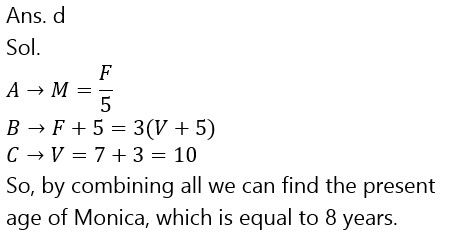1. What is the male population of the city?

A. The total population of the city is 40000.

B. If male population is increased by 4% the total population will be 41040.

C. If female population is increased by 10% the total population will be 41400.

(a) Only A and C

(b) Any two of them

(c) Only A and B

(d) All together are required

(e) Any one of them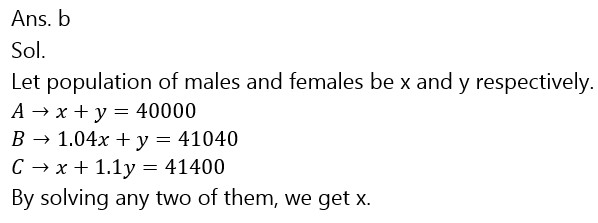1. What will be the average of three numbers

A. The largest no. is greater than the smallest by 20.

B. The sum of the largest and smallest numbers. is equal to twice the middle one.

C. The difference of the first two numbers is 10.

(a) Only A and C together

(b) Only B and C together

(c) Any two of them

(d) Question can’t be answered even after using all the statement

(e) All statements are required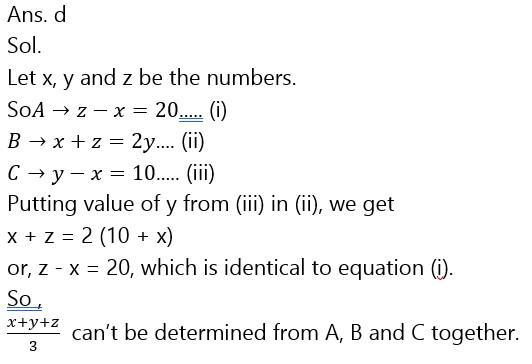1. A shopkeeper marks the price of an article and gives a discount of 20%. Find the marked price of the article.

A. If the discount is not given on the article then the shopkeeper gets 60% profit.

B. The cost price of the article is Rs 2500.

C. After giving the discount the shopkeeper gets 28% profit.

(a) Only A and B together

(b) Only B and C together

(c) Any two of them

(d) B and either A or C

(e) A and either B or C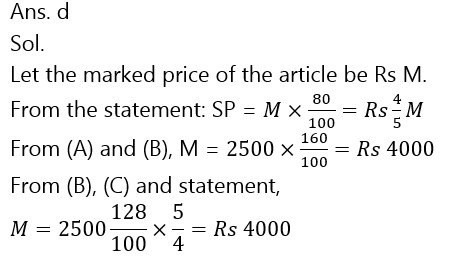1. In how many days can 32 workers complete a work?

A. 20% of the work can be completed by 16 workers in 4 days.

B. 64 workers can complete the work in 5 days.

C. One-fourth of the work is done by 20 workers in four days.

(a) Any two of them

(b) Only B

(c) Either A or C only

(d) A and C together

(e) Any one of the threeDirections (6-10): In the following pie-chart Income and expenses of five employees is given. Read the given data & answer the following questions.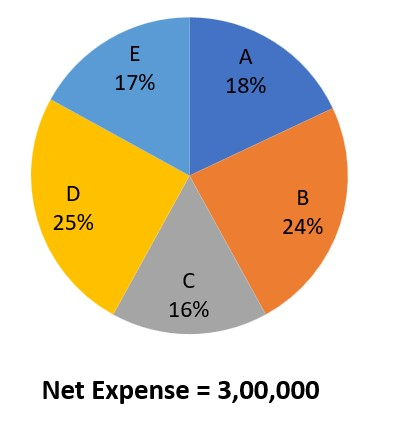1. What is the average saving of B and C ?

(a) 8,000

(b) 9,000
(c) 10,000

(d) 11,000

(e) 12,000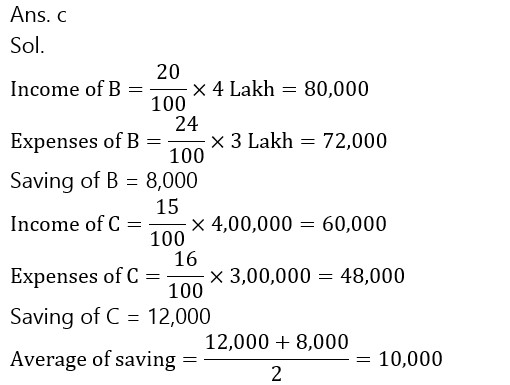1. If Income of Employee A decreases by 10% and its expenses increase by 20%. Then, his saving changes by what percent? (approximately)

(a) 42%

(b) 50%
(c) 58%

(d) 62%

(e) 64%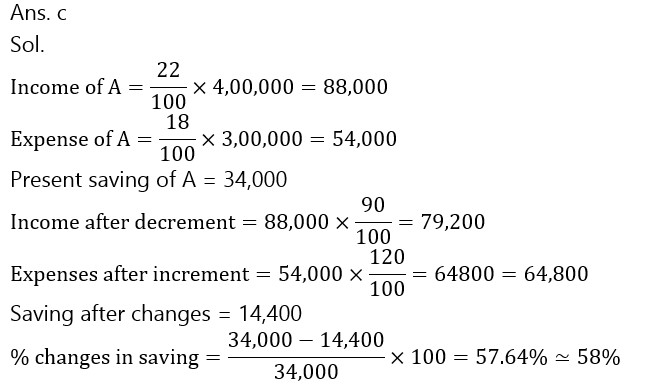1. If the income of D increases by 10% and income of E decreases by 20% then what will be the effect on net income of five employees ?

(a) Rs. 800 increase

(b) Rs. 1000 Increase
(c) Rs. 1000 decrease

(d) Rs. 800 decrease

(e) None of these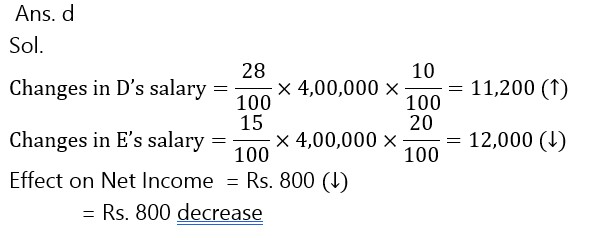1. What is the ratio between total saving of A to total saving of B ?

(a) 17 : 4

(b) 4 : 17
(c) 17 : 15

(d) 15 : 17

(e) 12 : 7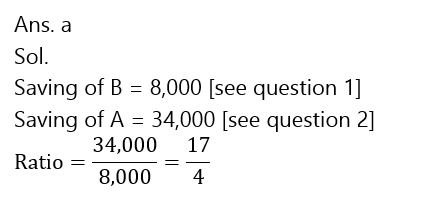1. If expenses of C increase by 15% then to how much percentage of increment is necessary in his income to keep his saving same as before ?

(a) 10%

(b) 12%
(c) 15%

(d) 8%

(e)16%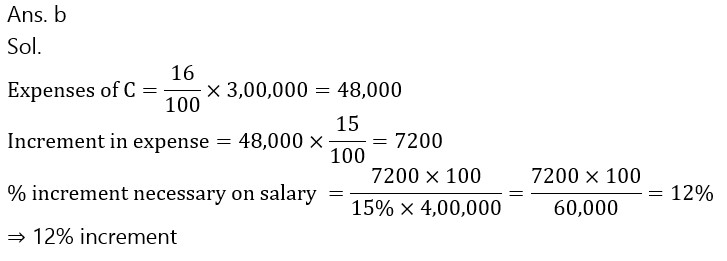### 2023 Preparation Kit PDF

#### Most important PDF’s for Bank, SSC, Railway and Other Government Exam : Download PDF Now

AATMA-NIRBHAR Series- Static GK/Awareness Practice Ebook PDF Get PDF here
The Banking Awareness 500 MCQs E-book| Bilingual (Hindi + English) Get PDF here
AATMA-NIRBHAR Series- Banking Awareness Practice Ebook PDF Get PDF here
Computer Awareness Capsule 2.O Get PDF here
AATMA-NIRBHAR Series Quantitative Aptitude Topic-Wise PDF 2020 Get PDF here
Memory Based Puzzle E-book | 2016-19 Exams Covered Get PDF here
Caselet Data Interpretation 200 Questions Get PDF here
Puzzle & Seating Arrangement E-Book for BANK PO MAINS (Vol-1) Get PDF here
ARITHMETIC DATA INTERPRETATION 2.O E-book Get PDF here

3